# 2. 算法基本原理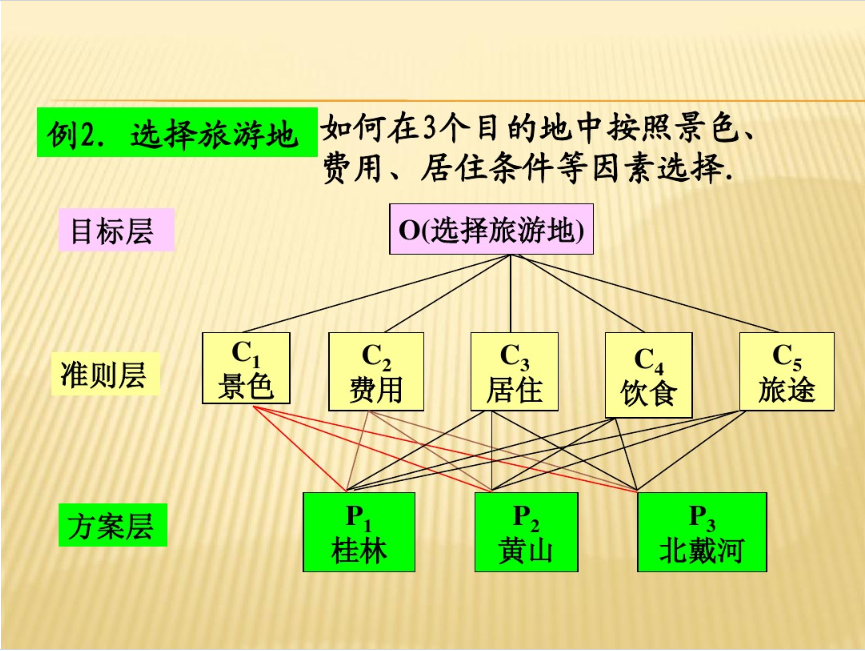## 2.2. 层次分析法的步骤

1.建立层次结构模型

• 将决策的目标、考虑的因素（决策准则）和决策对象按照他们之间的相互关系分为最高层、中间层和最低层，绘出层次结构图。
• 最高层： 决策的目的、要解决的问题。
最低层： 决策时的备选方案。
中间层： 考虑的因素、决策的准则。
• 对相邻的两层，称高层为目标层，低层为因素层

2.构造判断矩阵

1表示两个因素相比，具有同样重要性
3表示两个因素相比，一个因素比另一个因素稍微重要
5表示两个因素相比，一个因素比另一个因素明显重要
7表示两个因素相比，一个因素比另一个因素强烈重要
9表示两个因素相比，一个因素比另一个因素极端重要
2,4,6,8上述两相邻判断的中值

3.层次单排序及其一致性检验

C I = 0 CI=0 ,有完全的一致性；
C I CI 接近于0，有满意的一致性；
C I CI 越大，不一致越严重。

n1234567891011
RI000.580.901.121.241.321.411.451.491.51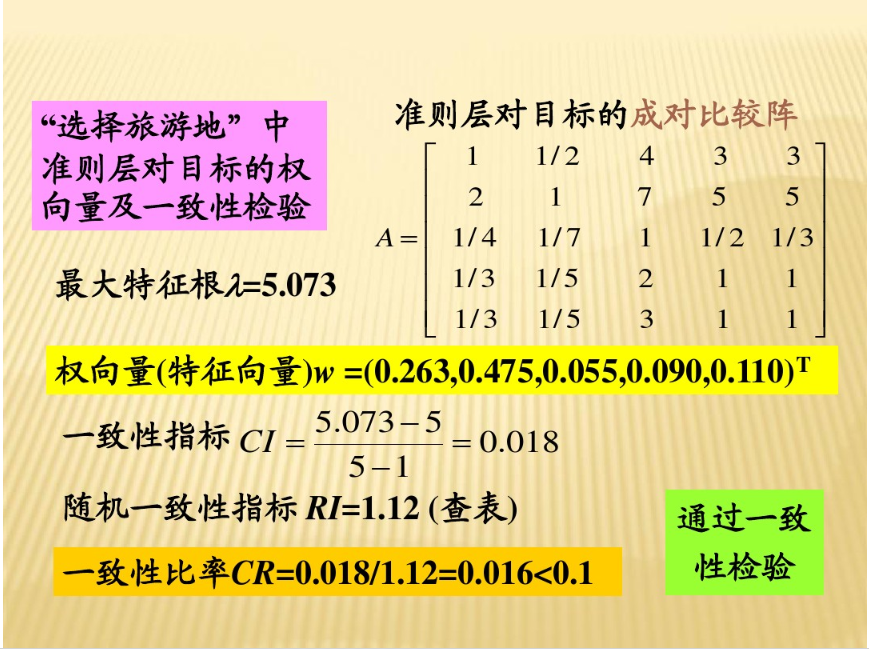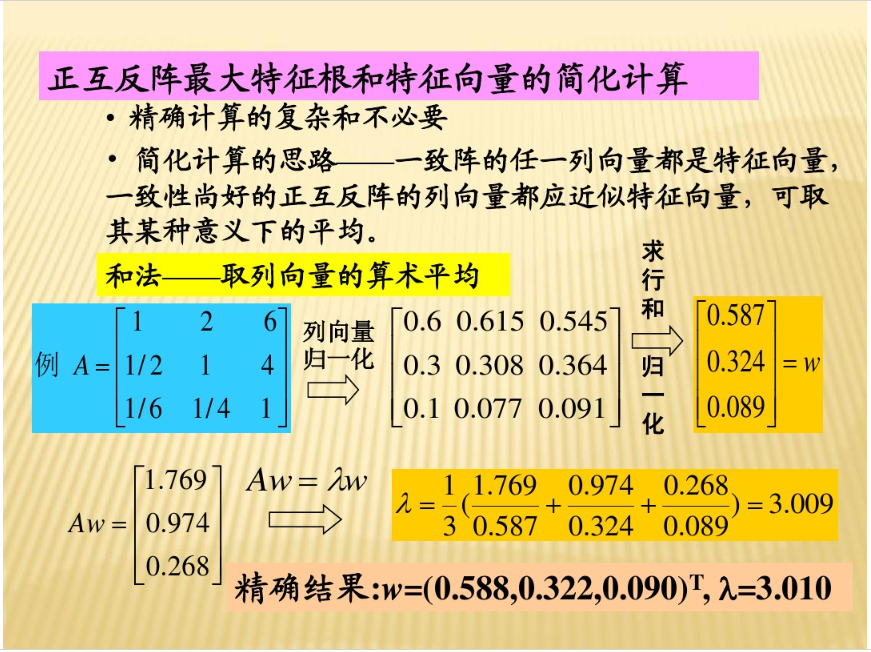4.层次总排序及其一致性检验

• 计算某一层次所有因素对于最高层（总目标）相对重要性的权值，称为层次总排序。
• 这一过程是从最高层次到最低层次依次进行的。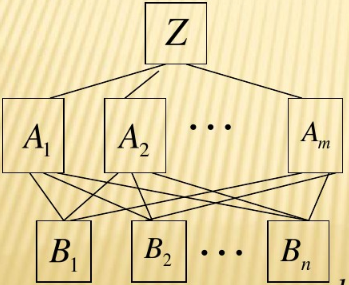A层 m m 个因素 A 1 , A 2 , ⋅ ⋅ ⋅ , A m , A_{1},A_{2},···,A_{m}, 对总目标Z的排序为 a 1 , a 2 , ⋅ ⋅ ⋅ , a m a_{1},a_{2},···,a_{m}
B层 n n 个因素对上层A中因素为 A j A_{j} 的层次单排序为 b 1 j , b 2 j , ⋅ ⋅ ⋅ , b n j ( j = 1 , 2 , 3 , ⋅ ⋅ ⋅ , m ) b_{1j},b_{2j},···,b_{nj}(j=1,2,3,···,m)

B层的层次总排序(即B层第 i i 个因素对总目标的权值为： ∑ j = 1 m a j b i j \sum_{j=1}^{m}a_{j}b_{ij} )为：
B 1 : a 1 b 11 + a 2 b 12 + ⋅ ⋅ ⋅ + a m b 1 m , B_{1}:a_{1}b_{11}+a_{2}b_{12}+···+a_{m}b_{1m},
B 2 : a 1 b 21 + a 2 b 22 + ⋅ ⋅ ⋅ + a m b 2 m , B_{2}:a_{1}b_{21}+a_{2}b_{22}+···+a_{m}b_{2m},
⋅ ⋅ ⋅ ···
B n : a 1 b n 1 + a 2 b n 2 + ⋅ ⋅ ⋅ + a m b n m , B_{n}:a_{1}b_{n1}+a_{2}b_{n2}+···+a_{m}b_{nm},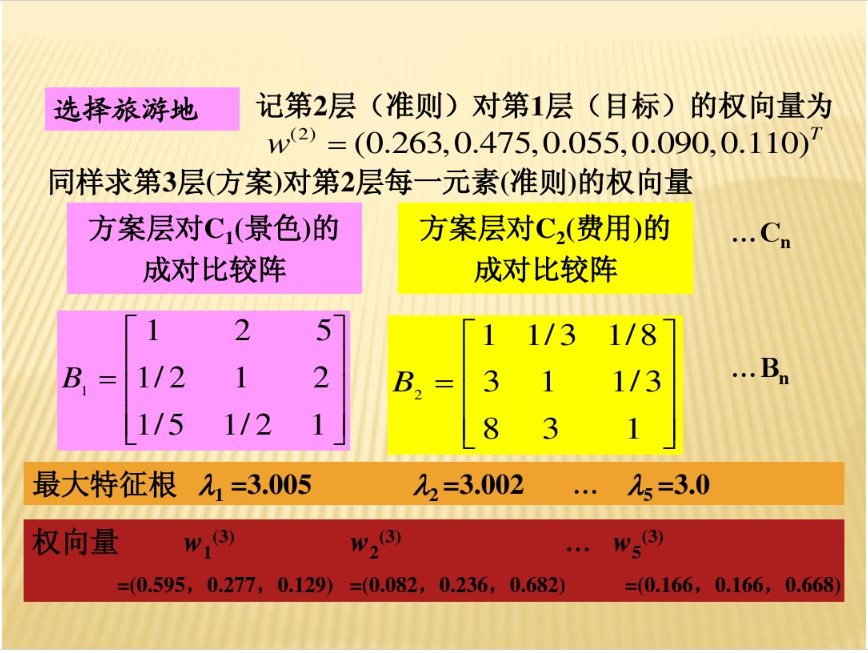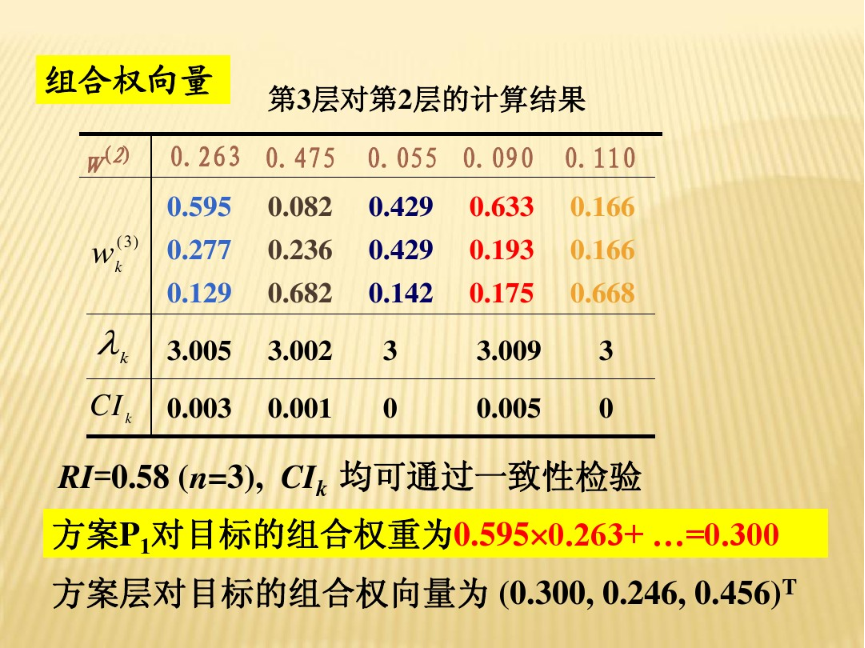# 3.算法总结

• 应用领域：经济计划个管理，能源政策和分配，人才选拔和评价，生产决策，交通运输，科研选题，产业结构，教育，医疗，环境，军事等。
• 处理问题类型：决策、评价、分析、预测等。
• 建立层次分析结构模型是关键一步，要有主要决策层参与。
• 构造成对比较矩阵是数量依据，应由经验丰富、判断力强的专家给出。

# 4.参考

1. 层次分析法建模——《百度文库》
05-15309204-176万+
08-307万+
01-061万+
02-217万+
08-262万+
07-082万+
10-15
01-101万+
©️2020 CSDN 皮肤主题: 大白 设计师:CSDN官方博客